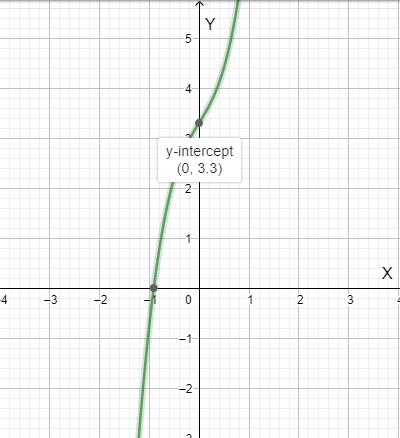# The given function is one to one function or not.### Precalculus: Mathematics for Calcu...

6th Edition
Stewart + 5 others
Publisher: Cengage Learning
ISBN: 9780840068071### Precalculus: Mathematics for Calcu...

6th Edition
Stewart + 5 others
Publisher: Cengage Learning
ISBN: 9780840068071

#### Solutions

Chapter 2, Problem 86RE
To determine

## To find: The given function is one to one function or not.

Expert Solution

The given function isn’t one to one.

### Explanation of Solution

Given function: q(x)=3.3+1.6x+2.5x3

Calculation: Use graphing device to graph the function q(x)=3.3+1.6x+2.5x3.

Graph : Sketch the graph using graphing utility.

Step 1: Press WINDOW button to access the Window editor.

Step 2: Press Y= button.

Step 3: Enter the expression 3.3+1.6x+2.5x3 which is required to graph.

Step 4: Press GRAPH button to graph the function and adjust the windows according to the graph.

The graph is obtained as:As we see that the graph is cutting the axis just one time. Therefore, the function is one to one function.

Hence, the function q(x) is one to one function.

Interpretation:

If some horizontal line intersects the graph of the function more than once, then the function is not one to one.

### Have a homework question?

Subscribe to bartleby learn! Ask subject matter experts 30 homework questions each month. Plus, you’ll have access to millions of step-by-step textbook answers!